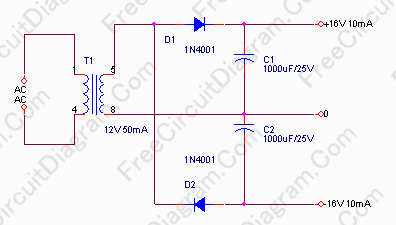## Multi Output Power Supply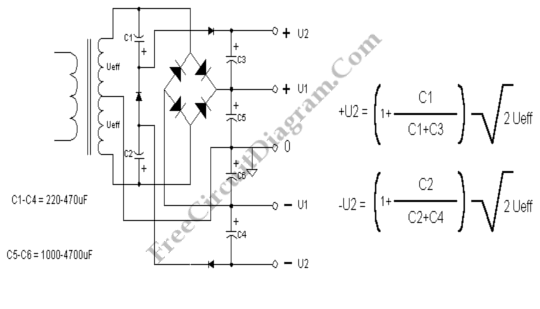This transformer-rectifier-filter arrangement gives you multiple output voltages with a single transformer. This AC-DC converter can be your solution is you need two level of symmetric power supply. Here is the schematic diagram of the circuit: U1 and -U1 uses a basic symmetric supply configuration, with a center-tapped transformer and full bridge rectifier for. U2 and -U2 uses a voltage […]

## Discrete Active Virtual Ground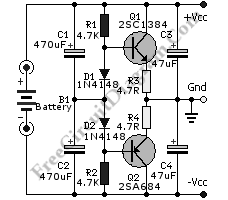You might find op-amp based active virtual ground, but here a  schematic diagram shows that you can build such circuit using discrete components.  This circuit uses most any complementary pair of small-signal transistors such as PN2222A and PN2907A. The diode that is used in this circuit is generic small-signal types like 1N914. You can also use other transistor type as […]

## Basic Symmetric Power Supply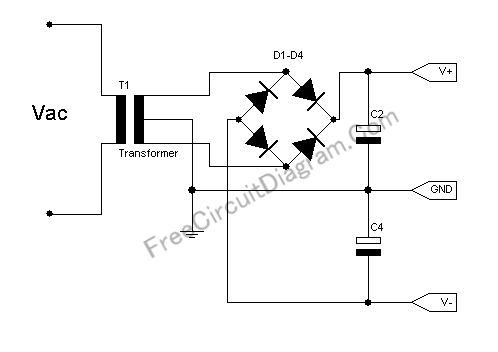The circuit below is the basic for standard symmetrical power supply. If your circuit need high current then this circuit is suitable. Here you can see the circuit’s schematic diagram. You need a center-tapped transformer to build this symmetric power supply. This power supply circuit is widely used in op-amp application, as well as in high-rated power amplifier. If you […]

## Regulated +15 -15 Volts Symmetric Power Supply From 12 Volt Transformer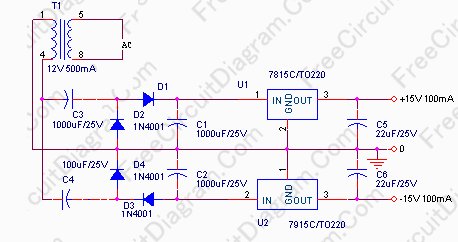If you need +-15 Volts supply and you have only a standard 12V AC transformer, don’t be hurry to buy a new one with center tap, you can use your old transformer! The schematic diagram of this circuit is shown in the figure below. The voltage before entering the regulator IC 7815 and 7915 might reach 24 volts, if there […]

## Another Symmetric Power Supply Without Center Tapped Transformer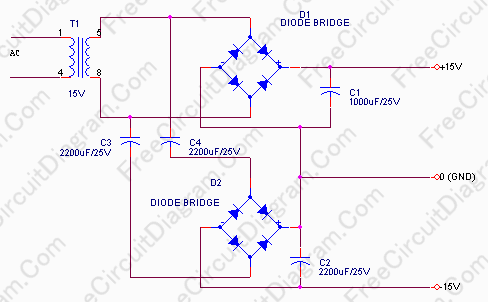Don’t worry if you need a symmetric power supply and you don’t have a center tapped transformer. The circuit below solve your problem. You can see the second bridge diode is powered via capacitor decoupling, so it’s DC isolated. Because it’s DC isolated, we can connect the ground together to form a symmetric supply. Because the second rectifier is powered […]